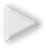Home

Episode 6 Supports

• Episode Description

Exploring: Sasha and Keoni use what they know about special points to explain why increasing the p-value results in the parabola getting wider on the coordinate grid.

• Focus Questions

For use in a classroom, pause the video and ask these questions:

1. [Pause video at 1:06]. What are Sasha and Keoni saying about the coordinates of a special point on the parabola? Do you agree? Why or why not?

2. [Pause video at 2:36]. Keoni says “it increases more.” What is increasing more?

• Supporting Dialogue

Provide opportunities for students to revoice a mathematical proposition. Ask a few students to revoice the proposition of this episode:

• Revoice Keoni and Sasha’s proposition that uses the special point to explain why increasing the p-value results in a wider parabola on the coordinate grid.

• Math Extensions

1. In this lesson, several arguments are made to justify why increasing the p-value results in a change in the width of the parabola. Write down each of these justifications.

2. How are the arguments similar? How are they different? Do you prefer one justification more than another? Explain.

•Mathematics in this LessonLesson Description

Targeted Understanding

CC Math Standards

CC Math Practices

Lesson Description

Sasha and Keoni use algebraic and geometric thinking to form three arguments that justify why a parabola gets wider on the coordinate grid as the p-value in y = x2/(4p) increases.

Targeted Understandings

This lesson can help students:

• Understand why, when the p-value in y =  x2/(4p) increases the parabolas appear to be getting wider on the same coordinate grid, by advancing three different arguments:

• When x is a fixed value (e.g., when x = 2 and y = 1/p, then as p increases, y decreases, which means the parabola will get wider.
• When y is a fixed value (e.g., when y = 4 and 4 = x2/(4p) or 4√p = x), then as p increases, the square root of p increases. This means that 4√p, which is x, also increases. Thus, as p increases, so does x, which means the parabola will get wider.
• A “special point” for a parabola (i.e., a point on the parabola that is aligned horizontally with the focus) can be expressed in general as (2p, p). Thus, as p increases, the special point for the parabola will be growing faster outward than it grows in the upward direction. This means the parabolas will appear to get wider on the same coordinate grid.

Common Core Math Standards

CCSS.M.HSF.IF.C.9: Compare properties of two functions each represented in a different way (algebraically, graphically, numerically in tables, or by verbal descriptions).

Sasha and Keoni first notice key features of the graphs of three quadratic functions (all with a vertex at the origin but with different p-values). For example, they notice that when the x-value is the same for all three functions (e.g., when x = 2), then the y-values of the points decrease as the distance between the vertex and focus (the p-value) increases. They observe visually on the graph how decreasing the y-value but keeping the x-value constant forces the parabola to become wider. They then link this observation to the algebraic expression for the parabolas. By fixing the x-value as 2, the equation y = x2/(4p) becomes y = 4/(4p), which is y = 1/p. Sasha and Keoni can see algebraically that as p increases, y decreases. Comparing the algebraic and graphical representations of these quadratic functions provides a way to help understand why increasing the p-value in y =  x2/(4p)  means the parabolas will appear to be getting wider on the same coordinate grid.

Common Core Math Practices

CCSS.Math.Practice.MP7. Look for and make use of structure.

In this lesson, Sasha and Keoni construct three different viable arguments to explain why  increasing the p-value in y = x2/(4p) means the parabolas will appear to be getting wider on the same coordinate grid. Each argument relies on a consideration of different features of the graphs: (a) fixing the x-value in Episodes 2-3; (b) fixing the y-value in Episode 4; and (c) considering special points in Episodes 5-6. Noticing a relationship is much easier than creating an argument to explain why the relationship holds! To accomplish the latter, Sasha and Keoni express their ideas to each other [Episode 2, 2:33 – 3:42], even when it’s difficult to do so. They also justify their conclusions [Episode 2, 5:02 – 5:33] and respond to each others’ explanations [Episode 6, 1:26 – 1:56].